Home > MC2 > Chapter 11 > Lesson 11.3.2 > Problem11-101

11-101.
1. Estimate each square root below to the nearest tenth. Then check your estimate on your calculator. Be sure to write down your estimate before you check! Homework Help ✎

1.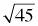2.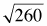3.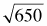4.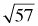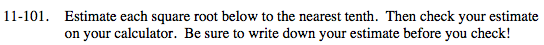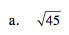The squares of what integers are near 45?

The square of 6 is 36, and thesquare of 7 is 49. The answer
must be between 6 and 7.

When you use your calculatorto check, you find that the
answer is approximately 6.7.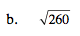See (a).

...122 = 144
132 = 169
142 = 196
152 = 225
162 = 256
172 = 289...

The answer must be between 16 and 17.

16.1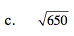See (a) and (b).

25.5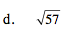See (a) and (b).